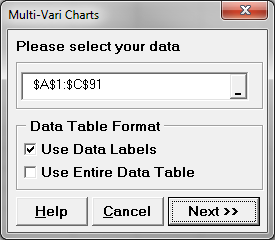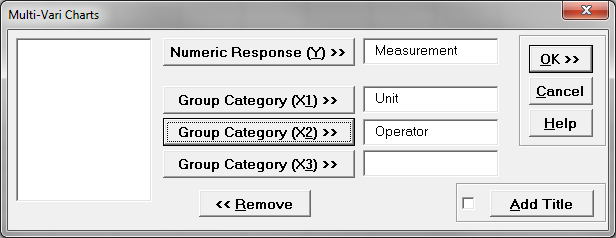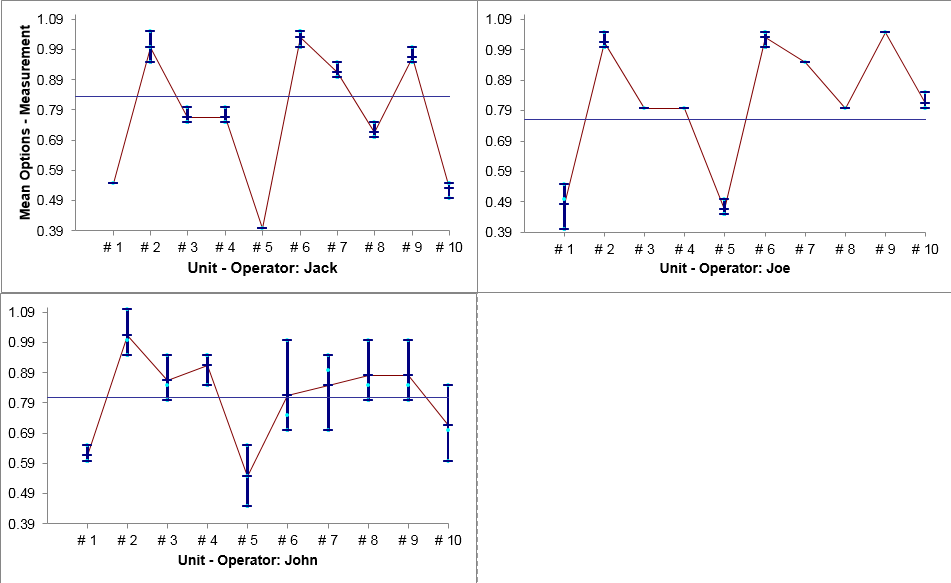Multi Vari Analysis with SigmaXL

Multi-Vari Analysis

Multi Vari Analysis is a graphic-driven method to analyze the effects of categorical inputs on a continuous output. It studies how the variation in the output changes across different inputs and helps us quantitatively determine the major source of variability in the output. Multi Vari charts are used to visualize the source of variation. They work for both crossed and nested hierarchies.

•  Y: continuous variable
•  X’s: discrete categorical variables. One X may have multiple levels

Hierarchy

Hierarchy is a structure of objects in which the relationship of objects can be expressed similar to an organization tree. Each object in the hierarchy is described as above, below, or at the same level as another one. If object A is above object B and they are directly connected to each other in the hierarchy tree, A is B’s parent and B is A’s child. In Multi-Vari analysis, we use the hierarchy to present the relationship between categorical factors (inputs). Each object in the hierarchy tree indicates a specific level of a factor (input). There are generally two types of hierarchies: crossed and nested.

• Crossed Hierarchy
In the hierarchy tree, if one child item has more than one parent item at the higher level, it is a crossed relationship.
• Nested Hierarchy
In the hierarchy tree, if one child item only has one parent item at the higher level, it is a nested relationship.

Use SigmaXL to Perform Multi Vari Analysis

Data File: “Multi-Vari” tab in “Sample Data.xlsx.”
Case study: ABC Company produces 10 kinds of units with different weights. Operators measure the weights of the units before sending them out to customers. Multiple factors could have an impact on the weight measurements. The ABC Company wants to have a better understanding of the main source of variability existing in the weight measurement. The ABC Company randomly selects three operators (Joe, John, and Jack) each of whom measures the weights of 10 different units. For each unit, there are three items sampled.

Steps in SigmaXL to perform a Multi-Vari Analysis:

1. Open the “Multi-Vari’ tab in “Sample Data.xlsx”
2. Select the range of data that we are interested in analyzing.
3. Click SigmaXL -> Graphical Tools -> Multi-Vari Charts
4. A new window also named “Multi-Vari Charts” pops up5. Click on “Next >>”
6. A new window also named “Multi-Vari Charts” pops up.
7. Select “Measurement” as “Numeric Response (Y)”
Select “Unit” as “Group Category (X1)”
Select “Operator” as “Group Category (X2)”8. Click “OK >>”Interpreting SigmaXL’s Multi-Vari Analysis

Model summary: Based on the Multi-Vari chart output, the measurement of units ranges from 0.4 to 1.1. Joe’s and John’s mean measurements stay between 0.8 and 0.9. Jack’s mean is slightly lower than both Joe’s and John’s. John has the worst variation when measuring the same kind of unit because John has the highest difference between the maximum and minimum bars for any kind of unit. By observing the black lines of three operators, it seems like all three operators’ measurements follow the same pattern. The operator-to-operator variability is not large. The unit-to-unit variability is large and it could be the main source of variation in measurements.